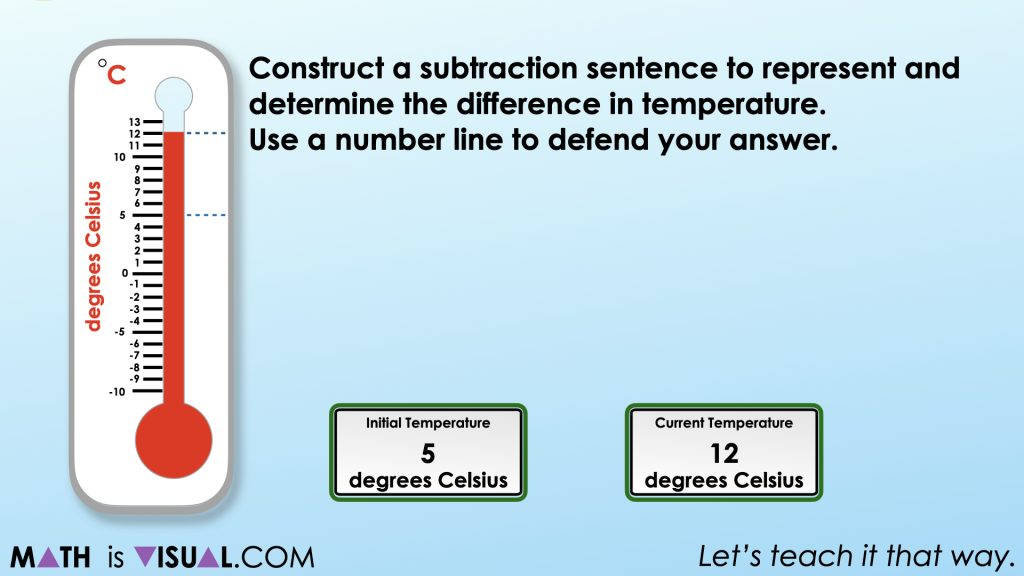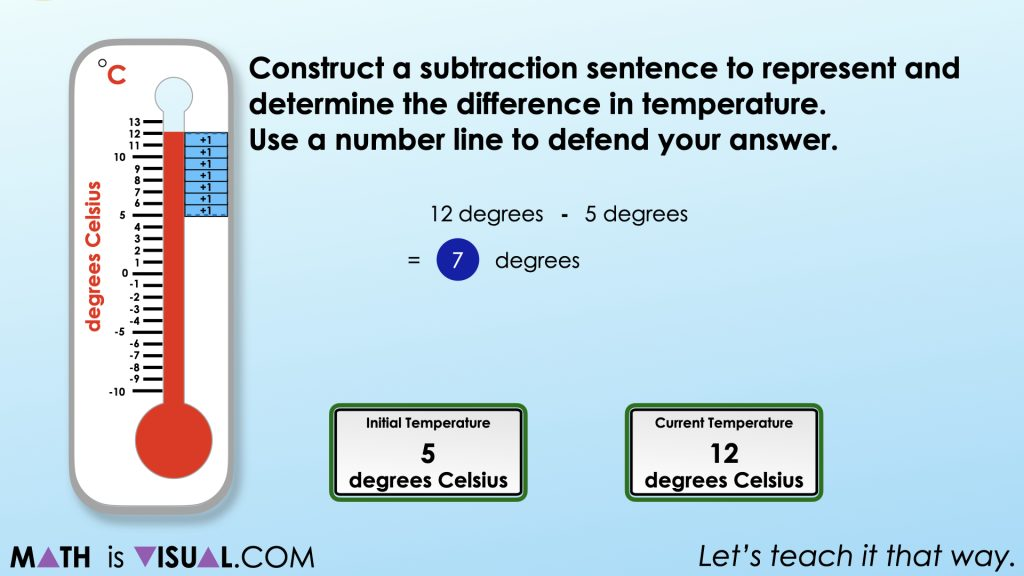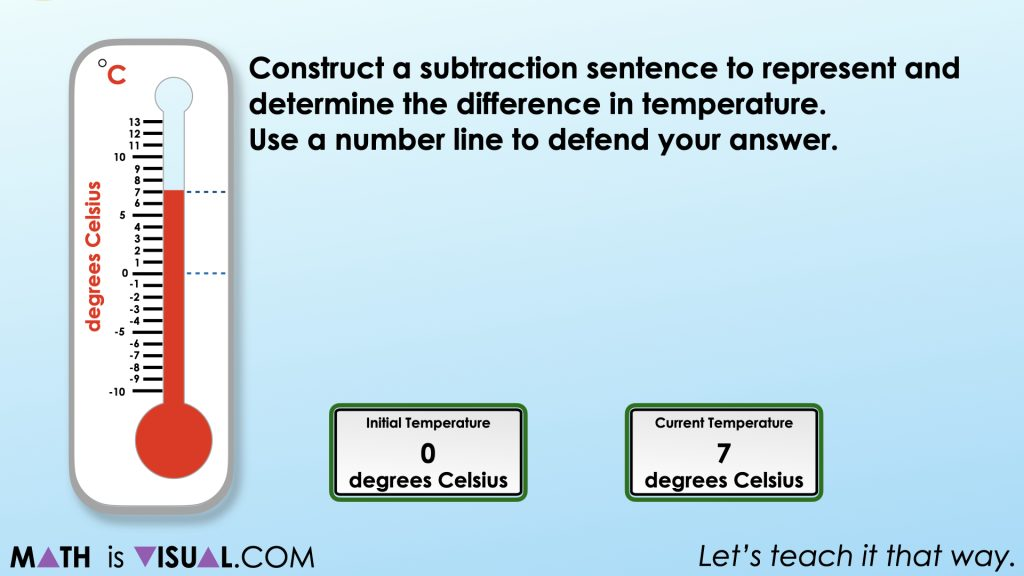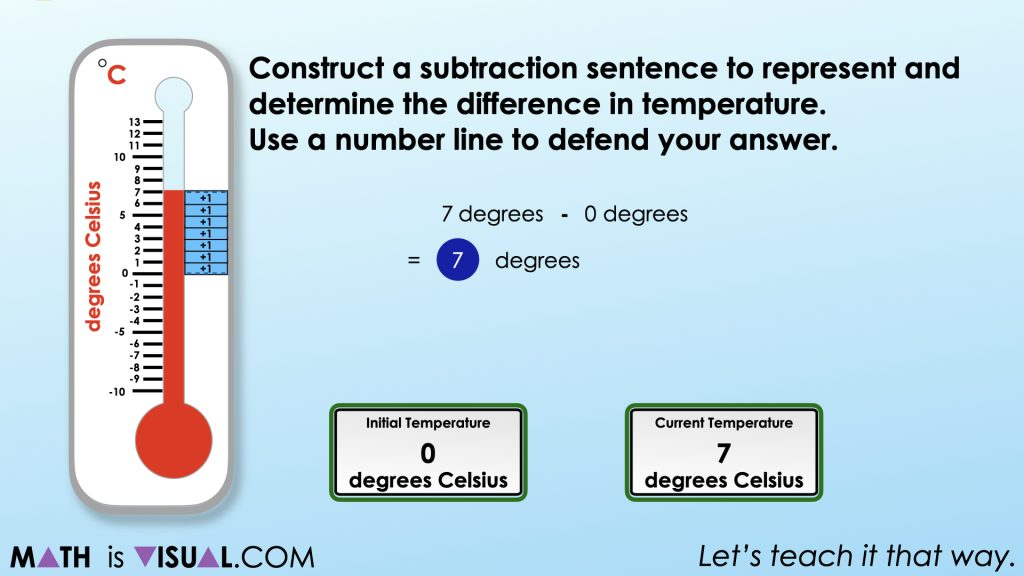# Subtracting Negative Numbers

Investigate subtracting negative numbers by finding the difference in temperature using the thermometer as a linear model number line.

## In This Set of Visual Number Talk Prompts…

Students will explore integer subtraction through a real world contextual situation involving rising and falling temperatures.

## String of Related Problems

Present the following subtraction sentences one at a time. Create a context that encourages students to leverage the subtraction structure of difference. For example, the temperature rose from 5 degrees to 12 degrees. How much warmer is it? The temperature rose from to -9 degrees to -2 degrees, how much warmer is it? Encourage students to use an open number line to defend their answer. Model student thinking on the open number line.

In this set of visual number talk prompts, we will explore the first two (2) of the five (5) related problems from Day 5 of the Rising Water problem based unit:

12 – 5

7 – 0

3 – (-4)

-2 – (-9)

-3 – (-10)

Facilitator note: The first four expressions are part of a string. Students will quickly realize that all four expressions have the same difference, they are simply adjusted down the number line by subtracting the same amount from both the minuend and the subtrahend.

The fifth equation is unique. Encourage students to adjust these values along the number line to defend their answer. For example, if I add 10 to both -3 and -10, my equation is 7 – 0.

## Visual Math Talk Prompt #1

Students will be prompted with:

Construct a subtraction sentence to represent and determine the difference in temperature. Use a number line to defend your answer.In this set of visual number talks, we give students yet another opportunity to wrestle with integer subtraction using the linear context of temperature.

As we did on Day 3 of this real world math unit, we begin with rising temperature from one positive temperature to another positive temperature resulting in a positive difference. Again, we want to model this using the number line despite most students firmly knowing and understanding the difference is 7 degrees Celsius in order to encourage the use of the tool for more challenging differences coming later in the string.## Visual Math Talk Prompt #2

Students will be prompted with:

Construct a subtraction sentence to represent and determine the difference in temperature. Use a number line to defend your answer.Again, students are given a very low floor opportunity to not only find the difference between a higher temperature and a lower temperature using the number line as a tool to represent their thinking.

It is important to model student thinking with the number line so that they are later able to leverage the number line as a tool for thinking when we begin working with negative temperatures.

It is worth noting that the difference between 7 degrees and 0 degrees is the same difference between 12 degrees and 5 degrees.Offer additional subtraction sentences where the difference is 7 degrees such as:

3 – (-4)

-2 – (-9)

-3 – (-10)

After this string, students should feel convinced that if the current temperature is greater than the initial temperature, the difference should be positive.

Can we generalize a rule for subtracting integers where the minuend is larger than the subtrahend?

## Want to Explore These Concepts & Skills Further?

Three (3) additional number talk prompts are available in Day 5 of the Rising Water problem based math unit that you can dive into now.

Why not start from the beginning of this contextual 6-day unit of real world lessons from the Make Math Moments Problem Based Units page.

Did you use this in your classroom or at home? How’d it go? Post in the comments!

Math IS Visual. Let’s teach it that way.